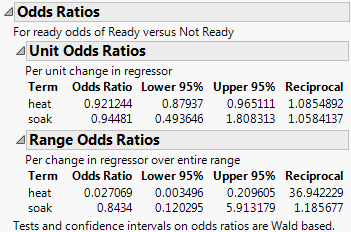Publication date: 05/24/2021

## Options for Nominal Fits

The following options are available in the Nominal Logistic Fit red triangle menu:

Plot Options

(Available only if the model consists of a single continuous effect.) The Plot Options menu contains the following options:

Show Points

Toggles the points in the Logistic Plot on or off.

Show Rate Curve

Enables you to compare the rate at various values of the effect variable with the fitted logistic curve. This option is useful only if you have several points for each value of the effect. In these cases, you get reasonable estimates of the rate at each value, and compare this rate with the fitted logistic curve. To prevent too many degenerate points, usually at zero or one, JMP shows only the rate value if there are at least three points at the x-value.

Line Color

Specifies the color of the plot curves.

Odds Ratios

Shows or hides an Odds Ratios report that contains Unit Odds Ratios and Range Odds Ratios.

Figure 11.6 Odds RatiosInverse Prediction

(Available only for two-level nominal responses.) Finds the x value that results in a specified probability. See the appendix Statistical Details for more information about inverse prediction.

Save Probability Formula

Creates columns in the current data table that contain formulas for linear combinations of the response levels, prediction formulas for the response levels, and a prediction formula giving the most likely response.

For a nominal response model with r levels, JMP creates the following columns:

columns called Lin[j] that contain a linear combination of the regressors for response levels j = 1, 2, ... r - 1

a column called Prob[r], with a formula for the fit to the last level, r

columns called Prob[j] for j < r with a formula for the fit to level j

a column called Most Likely response name that selects the most likely level of each row based on the computed probabilities.Publish Probability Formulas

Creates probability formulas and saves them as formula column scripts in the Formula Depot platform. If a Formula Depot report is not open, this option creates a Formula Depot report. See Formula Depot in Predictive and Specialized Modeling.

Indicator Parameterization Estimates

(Available only when there are nominal columns among the model effects.) Shows or hides the Indicator Function Parameterization report, which gives parameter estimates for the model where nominal columns are coded using indicator (SAS GLM) parameterization and are treated as continuous. Ordinal columns remain coded using the usual JMP coding scheme. The SAS GLM and JMP coding schemes are described in The Factor Models.

Caution: Standard errors and chi-square values given in the Indicator Function Parameterization report differ from those in the Parameter Estimates report. This is because the estimates are estimating different model parameters.